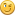## Help with Resource Cap Calculations Easimar Steel example

Started by Korda, May 21, 2022, 02:36 pm

#### Korda

##### May 21, 2022, 02:36 pm
I'm trying to work out a crafting calculation and having some issues. Can someone tell me where I'm going wrong?

I don't understand where the Easimar Steel score of 792.30 comes from in a Deepwell Capacitor.

Steel used in a Deepwell Capacitor has this weighting: CD=66% OQ=33%

With steel, the max CD is 650, for OQ it is 1000.

Here's my (wrong) calculation based on the Stat*1000/Maximum Cap formula
OQ = 377 *1000 / 1000 = 377 * .33 = 124
CD = 915, which is over the cap of 650 so its 650, which is 100% for the CD box. 1000 * .66 = 660

124 + 660 = 784, which does not match the value shown in SWGAide of 792.30
https://swgaide.com/schematics/view.php?server=138&schid=3740

Help me SWGAide, you're my only hope.

#### Mr-Miagi

##### May 21, 2022, 05:20 pm #1
Quote from: Korda on May 21, 2022, 02:36 pmI'm trying to work out a crafting calculation and having some issues. Can someone tell me where I'm going wrong?

I don't understand where the Easimar Steel score of 792.30 comes from in a Deepwell Capacitor.

Steel used in a Deepwell Capacitor has this weighting: CD=66% OQ=33%

With steel, the max CD is 650, for OQ it is 1000.

Here's my (wrong) calculation based on the Stat*1000/Maximum Cap formula
OQ = 377 *1000 / 1000 = 377 * .33 = 124
CD = 915, which is over the cap of 650 so its 650, which is 100% for the CD box. 1000 * .66 = 660

124 + 660 = 784, which does not match the value shown in SWGAide of 792.30
https://swgaide.com/schematics/view.php?server=138&schid=3740

Help me SWGAide, you're my only hope.

You need to use more digits to the right of the decimal place as 66% in decimal is pretty much .66666666  to infinity....  10 places would be enough to get the precision we need for swg.

`66 / 100 = .6666666666 # adjusted CD weight snipped for brevitynormally the formula would do this if cap was 1000(915 / 1000 * 1000) * .6666666666  = 609.9999999999 #However this steel CD caps at 650 thus the value used should be 1000 since that is the ceiling e.g:1000 * .6666666666 = 666.666666633 / 100 = .3333333333 # adjusted OQ weight snipped for brevity377 / 1000 * 1000) * .3333333333 = 125.6666667 # this steel OQ caps at 1000Next we simply add up the results to get rating:666.6666666 + 125.6666667 = 792.3333327`

#### Korda

##### May 21, 2022, 09:31 pm #2
Aha, thanks so much Mr-Miagi! I can't believe that accounted for so much disparity but it explains why the other formula I tested worked out fine.

Darned maths... I hate numbers.

Thanks againKorda

#### Mr-Miagi

##### May 25, 2022, 01:26 am #3
Quote from: Korda on May 21, 2022, 09:31 pmAha, thanks so much Mr-Miagi! I can't believe that accounted for so much disparity but it explains why the other formula I tested worked out fine.

Darned maths... I hate numbers.

Thanks againKorda

No problem! Don't feel bad, it's happened to me as well when using my old casio calculator in a quick determination, many of these calculators when you divide 66 into 100 give a 0.66  instead of filling the display. It's misleading if one is needing more precision.